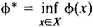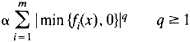# Penalty Function Method

## Penalty Function Method

a method of reducing problems of finding a relative extremum of a function.to problems of finding the absolute extremum.

An important application of the penalty function method is to problems of mathematical programming. Suppose we wish to minimize the function Φ(x) on the set X = {x: fi (x)≥ 0, i = 1, 2, . . ., m} of an n-dimensional Euclidean space. The function Ψ(x, α), which depends on x and on the numerical parameter a > 0, is called the penalty function (or penalty) for violation of the constraints fi (x)≥ 0, i = 1, 2, . . ., m. This functipn has the following properties: ψ(x,α) = 0 if xX and ψ(x, α) > 0 if xX. Let us construct the function M(x, α)= Φ(x) + ψ(x, α) and designate as x(α)any point of its absolute global minimum. LetThe function ψ(x, α) is selected so that ϕ(x(α)) → ϕ* as α → + ∞. The functionoften is selected as ψ(x, α). The selection of the specific form of the function ψ(x, α) involves both the problem of the convergence of the penalty function method and the problems that arise in solving the problem of absolute minimization of the function M(x, α).

In a somewhat more general formulation, the penalty function method consists in reducing the problem of minimizing the function ϕ(x) on a set X to the problem of minimizing some parametric function M(x, α) on a set whose structure is simpler, from the standpoint of effectiveness of the application of numerical methods of minimization, than that of X.

### REFERENCES

Moiseev, N. N. Elementy leorii optimal’nykh sistem. Moscow, 1975.
Fiacco, A., and G. McCormick. Nelineinoe programmirovanie. Moscow, 1972. (Translated from English.)
Céa, J. Optimizatsiia. Moscow, 1973. (Translated from French.)

V. G. KARMANOV

References in periodicals archive ?
In order to overcome the nonempty assumption of the interior of feasible region, Bian and Xue  proposed a recurrent neural network for nonsmooth convex optimization based on penalty function method.
Then a heuristic yet effective method, which combines the penalty function method and quasi-Newton method , is proposed to obtain a local solution iteratively.
When we use penalty function method in literature  to inverse optimization problem (7), the optimization problem (7) is turned into the following form by the method in literature :
Penalty function method is an important tool in solving nonlinear optimization problems.
Application of genetic algorithm and penalty function method in machine optimal design, Journal of China Jiliang University 15(4): 290-293.
Penalty function method is a technique which is used to solve the constrained optimization problems.
In constrained optimization problem, penalty function method has been adopted to transform problem into non-constrained ones.
Penalty function method (PFM) is utilized to solve constrained optimization problem, which can convert a constrained optimization problem into an unconstrained optimization problem .
They used a penalty function method and divided the constitutive equation into shear stress and normal stress components to decrease memory capacity.
Wang, "A novel penalty function method for semivectorial bilevel programming problem," Applied Mathematical Modelling.
Beyabanaki and Lin [7, 8] used an augmented Lagrange multiplier method instead of the penalty function method to address the contact issue in the DDA method, avoiding the difficulty of selecting the contact stiffness.
Site: Follow: Share:
Open / Close